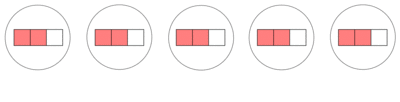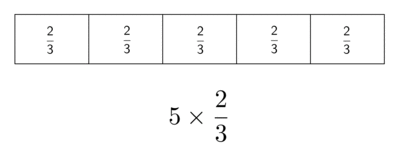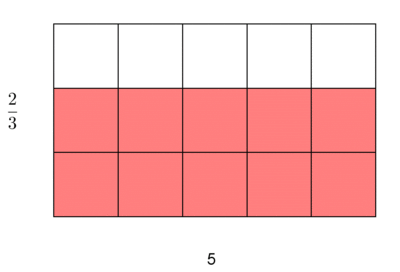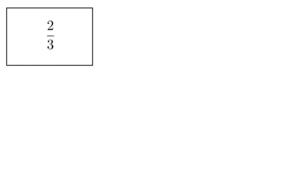# Extending Multiplication From Whole Numbers to Fractions

Alignments to Content Standards: 4.NF.B.4

1. Write a story problem that can be solved by finding $5\times4$.
2. Draw two different diagrams that show that $5\times4=20$. Explain how your diagrams represent $5\times4=20$.
3. Which of the diagrams you used to represent $5\times4=20$ can be used to represent $5\times\frac{2}{3}$? Draw the diagram if possible.

## IM Commentary

This task can be used at the beginning of a fourth grade unit on multiplication with fractions to evaluate what students know about multiplication. After knowing how students understand multiplication and the different diagrams they use, the teacher can better integrate their prior knowledge into future lessons about fraction multiplication.

For the first two questions, the goal is to see what students know about the meaning of multiplication based on how they represent multiplication with contexts and diagrams versus simply having strategies to solve multiplication problems. They may use a set representation, length representations such as a number line or a bar diagram, or a rectangle where the side lengths correspond to the factors and the area corresponds to the product. Understanding whether students have access to one or more of these representations is useful for planning future lessons. The teacher can sequence subsequent lessons to help students who only use a set representation to draw, e.g., a number line, and then see how that relates to the abstraction used in writing equations. The area representation will be especially useful for future work, especially when multiplying a fraction by a fraction.

The third question assesses whether students can extend multiplication of whole numbers to the case where one number is not a whole number. Some students may be very comfortable with this and can adapt their diagrams right away, while others may not know what to do at this point. The task is designed so that students who look for and make use of structure (MP 7) can move forward even if they have not seen or have forgotten how to extend the meaning of multiplication to this case.

This task can also be used as an instructional task where students work in groups and explain to partners and/or the class how their diagrams represent $5\times4$ and later $5\times\frac{2}{3}$. While working on the task, the students can also look for patterns in the diagrams that they use to represent products that include a fraction as a factor. Teacher questioning should push students to connect the meaning of multiplication to their diagrams and extending them to the case where one factor is a fraction, thus helping students to see and make use of structure (MP 7). When multiplying a whole number and a fraction ($a\times b$, where $a$ is a whole number and $b$ is a fraction) all of the diagrams mentioned above will work. However, the teacher should  focus especially on the length and area models because they will be most useful when it is time to think about the meaning of the product of two fractions.

This task was written as a part of a collaborative project between Illustrative Mathematics, the Smarter Balanced Digital Library, and the Teaching Channel.

## Solution

Part 1.

The student may create a context that uses the “groups of” representation, for example saying that there are five bags, and each bag has 4 apples. Together, there are 20 apples if you combine all of the five bags. Depending on the representations they choose, the student might also say that finding the area of a rectangle with side lengths 4 units and 5 units would be an appropriate context. Students may write a multiplicative comparison problem; for example, “Suzy has 4 lollipops and Lily has 5 times as many lollipops.”

Part 2.

Students might draw diagrams based on sets, length (such as a number line or bar diagrams) or area that represent equal groups. They also might draw a multiplicative comparison diagram. See examples below.

• The first diagram shows 5 groups of 4, which totals 20.

• The second diagram shows 5 rectangles of length 4 put end to end, which has a total length of 20.

• The third diagram shows a rectangle with side lengths 5 and 4 and area 20.

• The fourth diagram shows two rectangles; one is of length 4 and the other is 5 times as long with a total length of 20.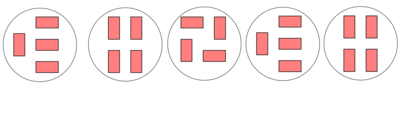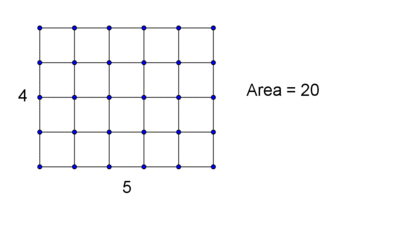Part 3.

All of diagrams can be adapted; see below.